# Topologically-Informed Atlas Learning

This work was accepted to ICRA 2022.

My friend Tommy Cohn led this project. Here's the project page he posted.

## Abstract

We present a new technique that enables manifold learning to accurately embed data manifolds that contain holes, without discarding any topological information. Manifold learning aims to embed high dimensional data into a lower dimensional Euclidean space by learning a coordinate chart, but it requires that the entire manifold can be embedded in a single chart. This is impossible for manifolds with holes. In such cases, it is necessary to learn an atlas: a collection of charts that collectively cover the entire manifold. We begin with many small charts, and combine them in a bottom-up approach, where charts are only combined if doing so will not introduce problematic topological features. When it is no longer possible to combine any charts, each chart is individually embedded with standard manifold learning techniques, completing the construction of the atlas. We show the efficacy of our method by constructing atlases for challenging synthetic manifolds; learning human motion embeddings from motion capture data; and learning kinematic models of articulated objects.

## A condensed explanation

If you aren't already familiar with the literature, I wonder how much you got from the abstract. Here's an attempt at a shorter, more visual explanation than the paper itself.

### Manifold learning

One of the most prevalent issues in robotics is the curse of dimensionality, in which statistical learning algorithms tend to become less effective as the number of dimensions in the data grows, thanks to the data becoming increasingly sparse.

The manifold hypothesis states that high-dimensional data often lies along low-dimensional manifolds, and has been demonstrated empirically. So naturally, one option when learning representations is to learn a representation that assumes the data lies along a manifold and then maps it to some low dimensional space.

Manifolds have the property that around every point, there exists a neighborhood homeomorphic to an n-dimensional Euclidean space. In simple terms, that just means that around every point on the manifold, you can think of the space as a Euclidean space; the below shows an example of this with a 3D manifold and 2D Euclidean space.

This particular property is important because manifold learning algorithms construct a mapping from the manifold to a lower-dimensional Euclidean space. Colloquially we refer to this as "unwrapping the manifold", as in the following depiction of data lying on the "swiss roll":

One can imagine that we could take any type of data that we assume lies on a manifold and unroll it cleanly to such a low dimensional representation.

### Atlas learning

A key observation about most manifold learning algorithms, such as ISOMAP, is that they attempt to unwrap manifolds in single pieces. However, some manifolds can't be unwrapped in this manner without cutting them, like the hollow sphere and the torus:

We say that these manifolds contain topological holes: the hollow sphere has enclosed space, whereas the torus has a hole formed by its inner loop. Manifolds with topological holes cannot be unwrapped in one piece, the way the swiss roll above was.

Instead, we turn to the mathematical definition of a manifold, which is given by coordinate charts that form an atlas. A coordinate chart is rigorously defined as a homeomorphism between a Euclidean neighborhood of the manifold and a subset of $\mathbb{R}^n$. Intuitively speaking, a coordinate chart can be described as a locally Euclidean region of the , like in the earlier figure where I highlighted one part of the manifold.

An atlas is a set of multiple coordinate charts that cover the manifold. Think of it like a globe that maps the world; you could cover it with a bunch of maps of smaller regions — the charts are the maps and the set of maps is the atlas. We want to do atlas learning, where we'd learn to construct a family of charts rather than a single mapping.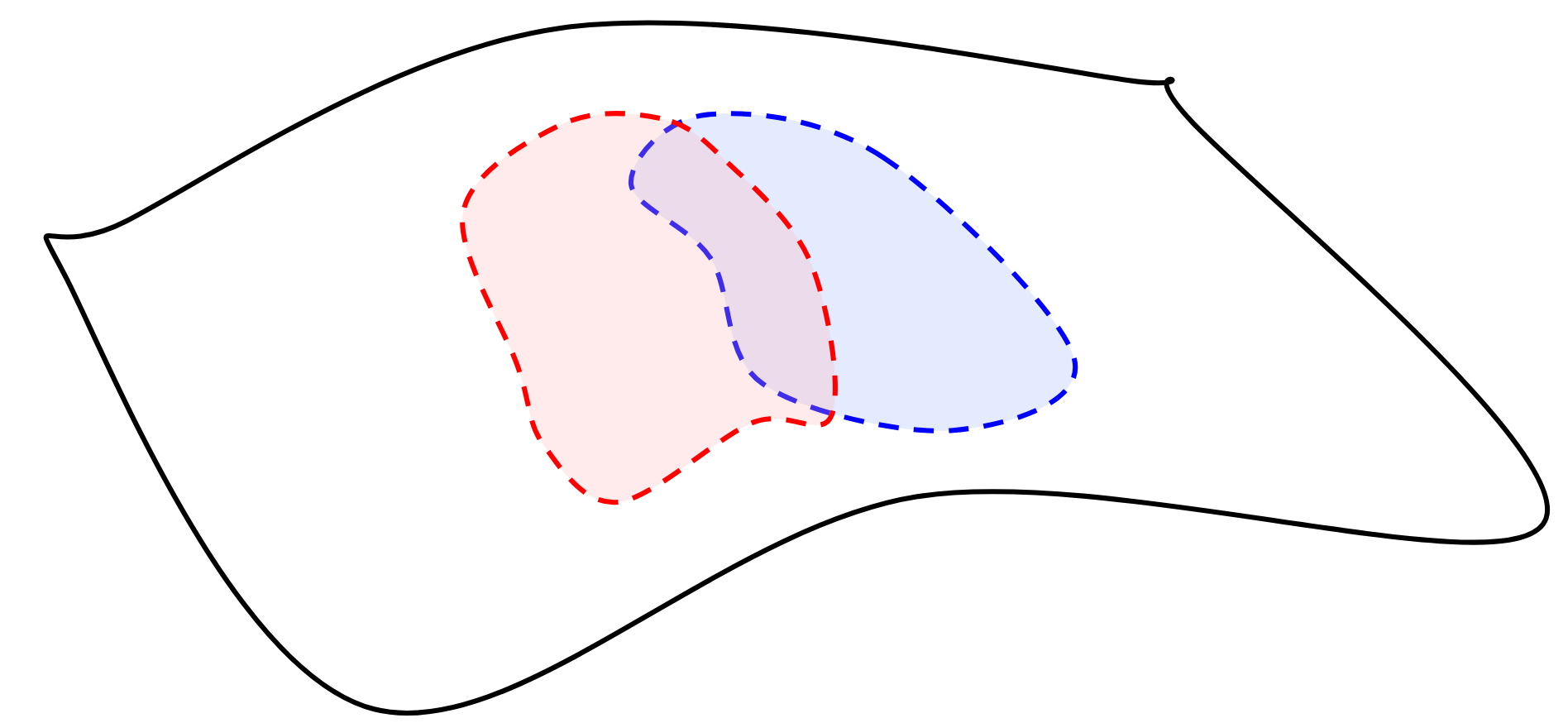An extremely simple example of an atlas with two charts that cover the manifold: blue and white.

The main contribution of our work was a method to do atlas learning that both creates nonlinear charts and . We demonstrated this method on different types of data that can't be unwrapped in a single manifold, which you'll see below.

### Overview of our method

Here's an overall description that we put on our poster, followed by explanations of the steps:

Step 1: Given some data, construct a neighborhood graph that connects data points to each other. The point of this is to initialize our manifold representation by describing the local geometry, so that our later chart search doesn't need to spend lots of time looking for this structure.

Forgive me here: our steps in this graphic are a bit inconsistent with the paper's numbered steps, so if you happen to be referencing this, here's a correction. Before combining charts, we need to initialize them. During initialization, we look to cover the manifold with a union of metric balls on the neighborhood graph (i.e. we start with some set of charts that's not necessarily efficient, but that fully encapsulates our data).

Step 2: Once we have an initial set of charts, we can combine them to end up with as small a set of charts as possible. We continually combine pairs of charts until no two charts can be combined. The criteria for whether two charts are able to be combined is if the intersection of these charts has a connected component, and if there are no atomic cycles in the intersection. Atomic cycles are cycles with no chords of a certain length, and indicate topological holes.

Here's a diagram showing an example of the intersection of two charts that cannot be combined:

Step 3: After combining charts, we embed each chart using the same method as ISOMAP. This embedding exists for each chart.

Step 4: If we want to get a datapoint by interpolating from embedding space, we construct an inverse mapping. This goes from the embedding space back to the original manifold; this is extremely useful for getting new data. For example, if we wanted to estimate the pose of an object and have some views of it mapped to embedding space, we could theoretically sample a new view via inverse mapping (we didn't actually do it for the example I'm giving here, but we have different applications instead!).

That's pretty much it! Behind all the topology and graph connectivity stuff it's actually a fairly simple method on its own. Applying this method is a bit harder depending on the data, which we'll show below.

### Qualitative results

If you want quantitative results, look at the paper. Here are some visual results.

First, we used our method on synthetic manifolds, namely the sphere and the torus: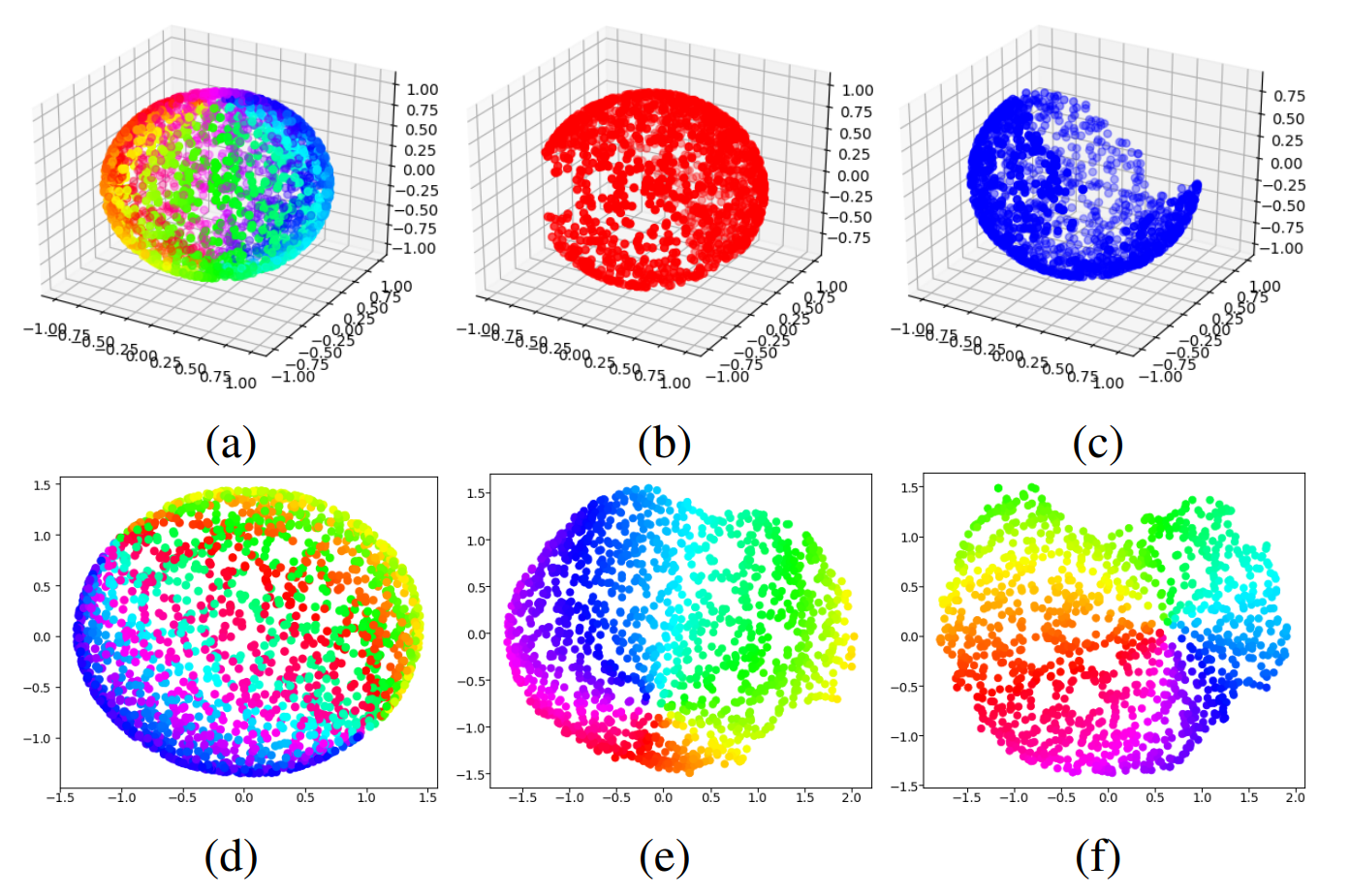Charts for a sphere. (a) is the original sphere, (b) and (c) are charts, (d) is an ISOMAP embedding of (a), (e) and (f) are respective ISOMAP embeddings of (b) and (c).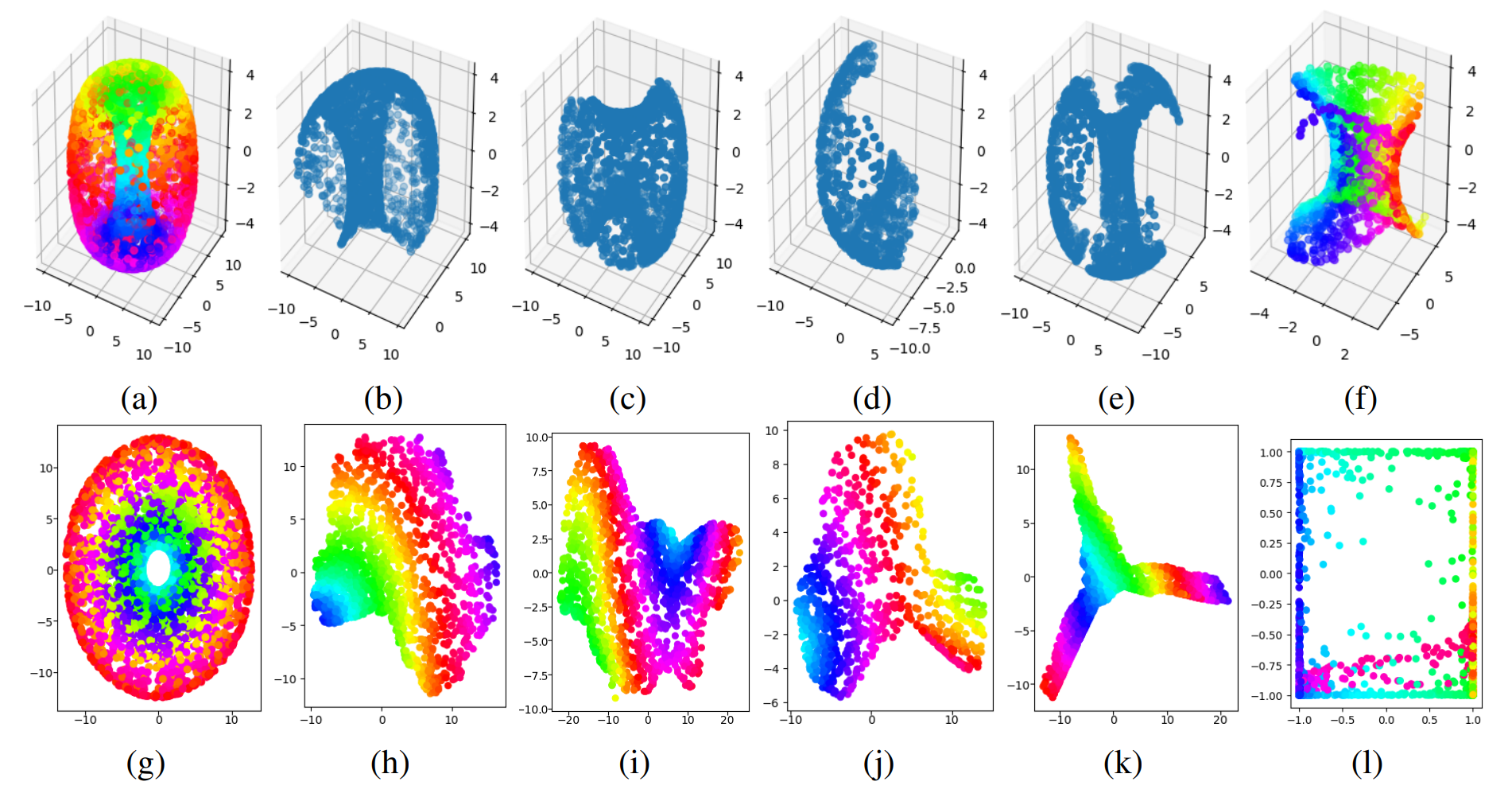Charts for a torus. (a) and (g) are the torus and ISOMAP embedding respectively, and (h)-(k) are embeddings of (b)-(e). (f) is an atlas created by a different autoencoder, for which its embedding (l) is shown to be inaccurate, as a comparison.

We also evaluated on the Klein bottle and SO(3) (yes, SO(3) is a smooth manifold!). We haven't produced visualizations of these though, so check the paper for quantitative results regarding them.

This was just to demonstrate that on the manifolds we motivated this problem with, they are indeed effective. More interesting to me, though, is data based on real world objects and motions.

One interesting type of data to us was human walking gait cycles, because these cycles are, uh, cyclic. Having cyclic data requires us to use atlas learning because of the present topological hole. We put this figure in the paper: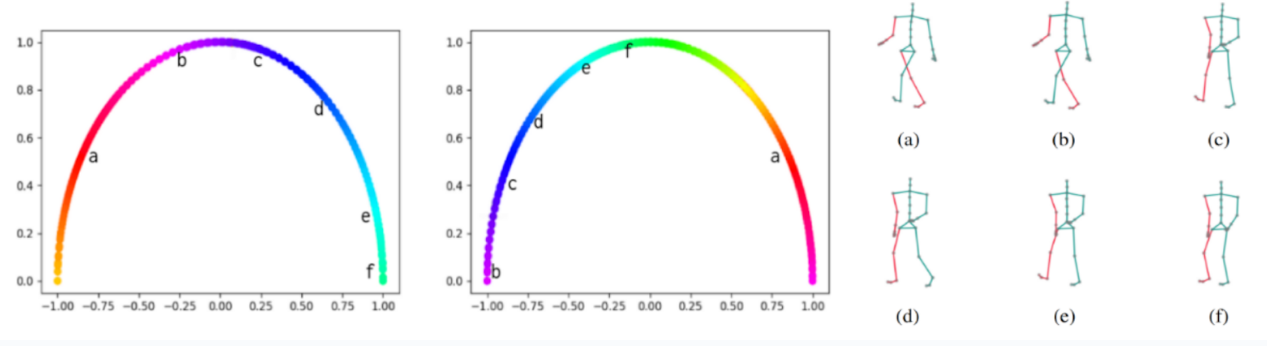Charts generated for walking poses from motion capture data. The letters in the charts denote embedding locations for the poses on the right.

We were able to map the space of poses in a specific walking trajectory using two charts, which is all we should theoretically need for a one-dimensional topological hole.

The next experiment we performed was based on custom data that we collected. We wanted to learn kinematic models for real objects, because oftentimes they have cyclic structure that needs to be accounted for in perception and manipulation tasks. The objects we used were a can opener and a corkscrew bottle opener.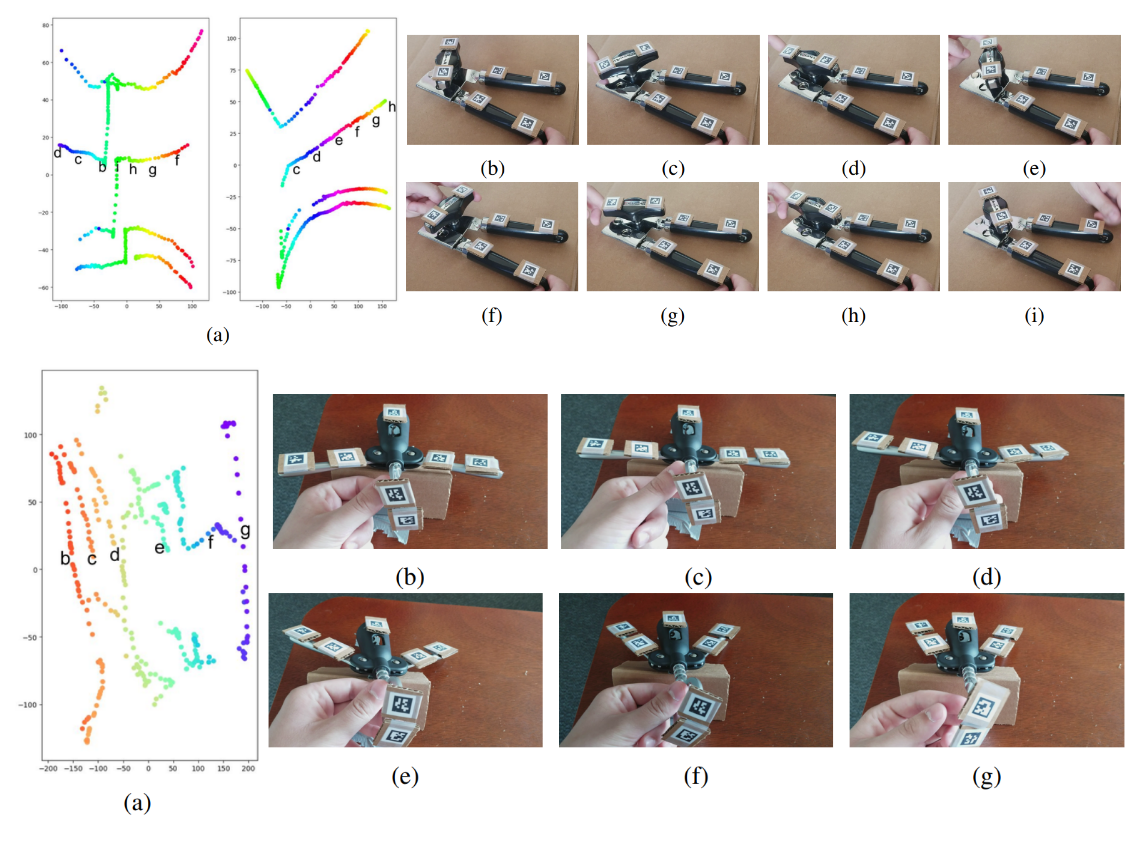Bottle opener (top) and can opener (bottom). The letters in the charts denote embedding locations for the poses on the right.

Here you'll notice that the embeddings are not quite clean thanks to the noisiness of using AprilTags. It was quite impressive to us that our method still generated reasonable embeddings that preserved topology in light of this, because it shows promise for real, unstructured

Our results show promise. But of course, there are many extended directions to take this project in.

## Extensions

These are a bunch of thoughts about some of the most interesting high-level ways in which this work could be extended.

One we proposed in the paper was that one could use alternative techniques to look for topological holes; in particular, the way we did this didn't guarantee that we'd find higher-dimensional holes in the cases that they occurred. We left that exploration as an open question.

Tommy recently started exploring an extension on his own, though I'm not sure if he intends to continue exploring it as of now. It had to do with minimizing the distortion of the learned representation (i.e. factor in geodesic distance on the manifold, get theoretical guarantees for both connectivity and similarity). You can ask him for more details about that.

Personally, though, I'm far more interested in the applications of methods like this. The walking gait cycle experiment was most exciting to me, because it got me thinking about locomotion: could we scale such a method to inferring poses for robot locomotion? Would this type of embedding make planning for walking easier, especially for learned controllers (example: learned gaits on Digit)? Similarly, applicability to perception and manipulation, as with the can opener, is also of great interest.

As with most academic work, however, applicability may suffer. Aside from more robust demonstrations, using this method might be difficult as is in a real setting, because one issue was visualization; it's very important when developing learning algorithms that practitioners are able to intuitively understand their outputs. In the manifold learning case, visualizing a manifold and its charts autonomously would be extremely helpful. However, constructing these visualizations was an effort in and of itself. For example, for the walking gait cycle visualization, because we already were aware of the cyclic nature of the data, we were able to manually parameterize the embedding to plot in a half circle; the default plot would be less intuitive (like the can/bottle opener plots). This boils down to intuitively knowing the structure of your data before trying to visualize it, which is a whole problem on its own.

Anyway, it's also difficult to imagine how to cleanly incorporate an atlas learning method into an existing perceptual system. One of the directions I've thought about that could make this easier is making this process fully differentiable. Differentiability is particularly important if we're to follow the trend of incorporating arbitrary parts of the optimization process into a learning pipeline (see: differentiable physics simulators). Fully differentiable atlas learning could be one way to seamlessly incorporate this into existing neural network pipelines. I could ramble about differentiable programming, but I'll shelve that discussion for now — would be interested in talking further about this topic though.

Finally, one of the things that would've made , but that we didn't really have the time for before the ICRA deadline, was seeing whether these learned embeddings could be incorporated into real robot plans. For example, could we get a dexterous manipulator to twist a corkscrew bottle opener to open a bottle properly, solely from learning interactions with articulated objects? That's a lot of effort and might warrant another paper of its own, but that would be a great natural demonstration of our method working on a real robot. I don't have any plans of pursuing this, but it's worth a shot for anyone who wants to.